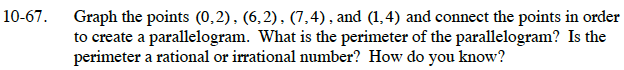Home > ACC7 > Chapter cc310 > Lesson cc310.1.5 > Problem10-67

10-67.

Graph the points (0, 2), (6, 2), (7, 4), (1, 4) and connect the points in order to create a parallelogram. What is the perimeter of the parallelogram? Is the perimeter a rational or irrational number? How do you know? 10-67 HW eTool (Desmos). Homework Help ✎In order to find the perimeter, you need to know the lengths of all 4 sides of the parallelogram. You know two of the sides from the graph. How can you find the lengths of the other two sides?

Draw a triangle so that the missing side is the hypotenuse of the triangle. Then use the Pythagorean Theorem to find the length of the hypotenuse.

1² + 2² = hypotenuse²
1 + 4 = hypotenuse²
5 = hypotenuse²

$\text{hypotenuse }=\; \sqrt{5}$

Now add all the sides together to get the perimeter of the parallelogram.

Does the answer for the perimeter repeat or terminate?

Use the eTool below to graph the points and create a parallelogram.
Click the link at right for the full version of the eTool: CC3 10-67 HW eTool .